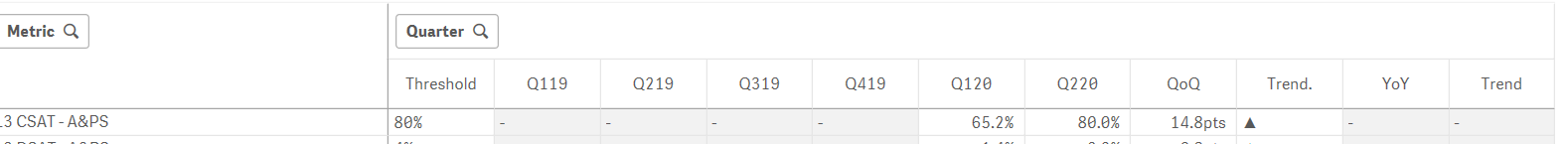# New to Qlik Sense

If you’re new to Qlik Sense, start with this Discussion Board and get up-to-speed quickly.

Announcements
Our May 2021 end-to-end product release from Data Integration to Data Analytics is out! READ DETAILS
cancel
Showing results for
Search instead for
Did you mean:Contributor III

## Completed Quarter values

HI,

In my Qlik Sense Report i am writing some expression as below.

i have categorized all percentage metrics into one flag and all numerical volumes into another flag as per below

the 2 variables i wrote for rolling Quarters

vMinMonth

vGetMaxQuarterMonthFinal

My Quarter values is always sum of 3 months

now what happening is when it comes to quarter ending last month

out of 10 Metrics  8 metrics data will come in time and under Q220  ----- so no problem it will give sum of 3 months Number.

but remaining 2 metrics data for last month of Quarter will be delayed .

So , it creates confusion to user's as they are thinking its complete Quarter number and using the QTD Number.

So now what i need is unless it has Sum of 3 months data i should not see the value for that metric under Q220  as per below snapshot

how can i write ?If ( MAX ( {<ScenarioLabel={'Actuals'},Scope={'APS'},Month={">=\$(vMinMonth) <=\$(vGetMaxQuarterMonthFinal)"}>} APSMetric_Flag ) = 1,

num ( SUM ({<ScenarioLabel={'Actuals'},Month={">=\$(vMinMonth) <=\$(vGetMaxQuarterMonthFinal)"},Scope={'APS'}>} NumValue) /
SUM ({<ScenarioLabel={'Actuals'},Month={">=\$(vMinMonth) <=\$(vGetMaxQuarterMonthFinal)"},Scope={'APS'}>} DenValue), '0.#%')

/////////////////////////////////////////////////////////////////////////////////////////////////////////////////////////////////////

If ( MAX ( {<ScenarioLabel={'Actuals'},Scope={'APS'},Month={">=\$(vMinMonth) <=\$(vGetMaxQuarterMonthFinal)"}>} APSMetric_Flag ) = 2,

num ( SUM ({<ScenarioLabel={'Actuals'},Month={">=\$(vMinMonth) <=\$(vGetMaxQuarterMonthFinal)"},Scope={'APS'}>} NumValue),'##,###.0')

0 Replies